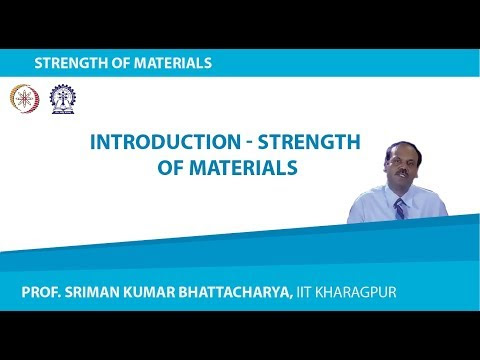### Strength of MaterialsAnalysis of stresses
Body forces, Surface forces, Internal Force, Stress at a point, Components of stress in Rectangular coordinates, Stress tensor, Principal stresses, Transformation, Equations, Stress invariants, Plane stress, Mohr’s circle for plane stress, Octahedral stresses, Differential equations of equilibrium, Components of stresses in cylindrical and Polar coordinates,
Analysis of Strain
Deformable bodies, Concepts of normal strain and shear strain, Strain components at a point, Transformation equations, Principal strains, Mohr’s circle of strains, Compatibility conditions, Displacement equation of equililibirum, Plane strain.
Stress-Strain relations
Uniaxial tensile test, Elasticity, Anelasticity , Work-hardening, anisotropy, homogeneity and continuity, generalized Hooke’s law, Lame’s constants, Modulus of rigidity, Bulk modulus, relation between the elastic constants, Principle of superposition, Uniqueness theorem, Thermal effects.
Bars of variable cross-section, Statically indeterminate problems in a tension and compression, Thin cylindrical and spherical vessels.
Torsion
Geometry of deformation of a twisted circular shaft, Stress and deformation in twisted circular solid and hollow shafts, Strain energy due to torsion , Power transmitted by circular shafts.
Bending of Beams
Bending moment and shear force diagrams, Stresses due to bending, bending equation, shear stresses in symmetrical elastic beams transmitting both shear and bending moment.
Deflection of Beams
The moment curvature relation, Macaulay’s and moment-area method, Castigliano’s theorem.
Combined Stresses
Beam subjected to bending and shear, shaft subjected to bending and torsion, short columns.
Stability of Columns
Stable and unstable equilibrium, Euler’s formula for long columns, Rankine’s formula.
Springs
Types of Springs. Close coiled and open coiled springs